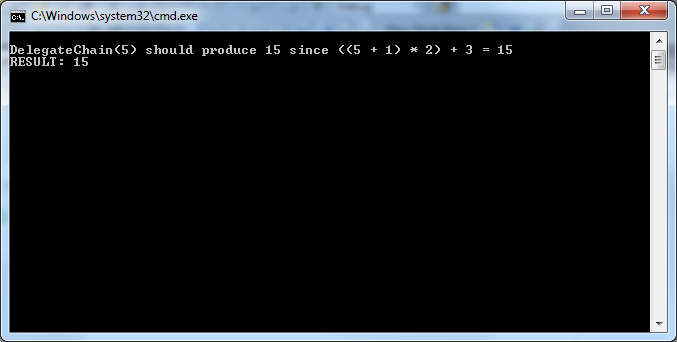# Lambda Expressions and Delegates in C#

In a previous post I discussed chaining C# delegates together. In the source code example, I created a generic DelegateMath class to house a few basic math operations. This time we will replace those functions with simpler and shorter lambda expressions.

So what exactly is a lambda expression? What does it have to do with C#? Our friends over at MSDN have this to say:

A lambda expression is an anonymous function that can contain expressions and statements, and can be used to create delegates or expression tree types.

All lambda expressions use the lambda operator =>, which is read as “goes to”. The left side of the lambda operator specifies the input parameters (if any) and the right side holds the expression or statement block. The lambda expression x => x * x is read “x goes to x times x.”

If we wanted to build a simple delegate using a lambda expression, it could look something like this:

``````delegate int del(int i);
del ADelegate = x => x * x;
int j = ADelegate(5); // j = 25
``````

So why use lambda expression with delegates at all? First of all they can be used anywhere an anonymous delegate can be used. The defining characteristic of lambda expressions is that they can be used with expression trees (which can then be used for LINQ and SQL purposes) while anonymous delegates cannot be used with expression trees.

If we take the same example from the previous post using delegates, we can modify it to use lambda expressions instead…

``````using System;

namespace DelegateChainWithLambda
{
// Declare the delegate type
delegate void UrdaDelegate(ref int x);

public class DelegateChainExampleWithLambda
{
public static void Main(string[] args)
{
// Create delegate objects from lambda expressions.
UrdaDelegate delegate01 = (ref int a) => { a = a + 1; };
UrdaDelegate delegate02 = (ref int b) => { b = b * 2; };
UrdaDelegate delegate03 = (ref int c) => { c = c + 3; };

// Chain the delegates together. The variable manipulation will
// start with delegate01, then delegate02, and end with delegate03.
UrdaDelegate DelegateChain = delegate01 + delegate02 + delegate03;

// Define our value, and build the expected result from it
int value = 5;
int expected = ((value + 1) * 2) + 3;

// Build a string for explaining the output
string ExplanationString = "\n";
ExplanationString += "DelegateChain(5) should produce " + expected;
ExplanationString += " since ((" + value + " + 1) * 2) + 3 = ";
ExplanationString += expected;

// Pass the value into the delegate chain
DelegateChain(ref value);

// ...and write the explanation and result to console!
Console.WriteLine(ExplanationString);
Console.WriteLine("RESULT: " + value + "\n");
}
}
}
``````

…and when we run the program we get this output:We still get the exact same output (cool story, I know) however we have removed the need for the math class, and made the code a little easier to follow.

Written on August 27, 2010